# Test: Process- 1

Test Description

## 20 Questions MCQ Test GATE Computer Science Engineering(CSE) 2023 Mock Test Series | Test: Process- 1

Test: Process- 1 for Computer Science Engineering (CSE) 2023 is part of GATE Computer Science Engineering(CSE) 2023 Mock Test Series preparation. The Test: Process- 1 questions and answers have been prepared according to the Computer Science Engineering (CSE) exam syllabus.The Test: Process- 1 MCQs are made for Computer Science Engineering (CSE) 2023 Exam. Find important definitions, questions, notes, meanings, examples, exercises, MCQs and online tests for Test: Process- 1 below.
Solutions of Test: Process- 1 questions in English are available as part of our GATE Computer Science Engineering(CSE) 2023 Mock Test Series for Computer Science Engineering (CSE) & Test: Process- 1 solutions in Hindi for GATE Computer Science Engineering(CSE) 2023 Mock Test Series course. Download more important topics, notes, lectures and mock test series for Computer Science Engineering (CSE) Exam by signing up for free. Attempt Test: Process- 1 | 20 questions in 60 minutes | Mock test for Computer Science Engineering (CSE) preparation | Free important questions MCQ to study GATE Computer Science Engineering(CSE) 2023 Mock Test Series for Computer Science Engineering (CSE) Exam | Download free PDF with solutions
 1 Crore+ students have signed up on EduRev. Have you?
Test: Process- 1 - Question 1

### Which one of the following is FALSE?

Detailed Solution for Test: Process- 1 - Question 1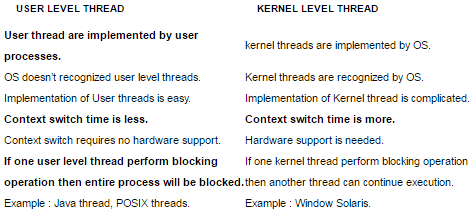Test: Process- 1 - Question 2

### Consider two processors P1 and P2 executing the same instruction set. Assume that under identical conditions, for the same input, a program running on P2 takes 25% less time but incurs 20% more CPI (clock cycles per instruction) as compared to the program running on P1. If the clock frequency of P1 is 1GHz, then the clock frequency of P2 (in GHz) is _________.

Detailed Solution for Test: Process- 1 - Question 2

► For P1 clock period = 1ns Let clock period for P2 be t.
► Now consider following equation based on specification 7.5 ns = 12*t ns
We get t and inverse of t will be 1.6GHz

Test: Process- 1 - Question 3

### Consider the procedure below for the Producer-Consumer problem which uses semaphores: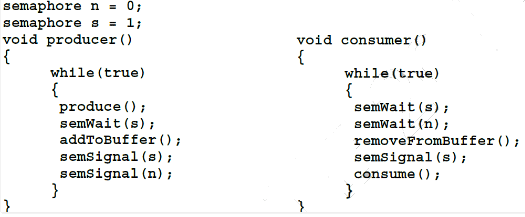Q. Which one of the following is TRUE?

Detailed Solution for Test: Process- 1 - Question 3

Initially, there is no element in the buffer.
► Semaphore s = 1 and semaphore n = 0.
We assume that initially control goes to the consumer when buffer is empty.
semWait(s) decrements the value of semaphore ‘s’ . Now, s = 0 and semWait(n) decrements the value of semaphore ‘n’. Since, the value of semaphore ‘n’ becomes less than 0 , the control stucks in while loop of function semWait() and a deadlock arises.

Thus, deadlock occurs if the consumer succeeds in acquiring semaphore s when the buffer is empty.

Test: Process- 1 - Question 4

The atomic fetch-and-set x, y instruction unconditionally sets the memory location x to 1 and fetches the old value of x n y without allowing any intervening access to the memory location x. consider the following implementation of P and V functions on a binary semaphore S.

void P (binary_semaphore *s)
{
unsigned y;
unsigned *x = &(s->value);
do
{
fetch-and-set x, y;
}
while (y);
}
void V (binary_semaphore *s)
{
S->value = 0;
}

Q. Which one of the following is true?

Detailed Solution for Test: Process- 1 - Question 4

Let us talk about the operation P(). It stores the value of s in x, then it fetches the old value of x, stores it in y and sets x as 1. The while loop of a process will continue forever if some other process doesn’t execute V() and sets the value of s as 0. If context switching is disabled in P, the while loop will run forever as no other process will be able to execute V().

Test: Process- 1 - Question 5

Barrier is a synchronization construct where a set of processes synchronizes globally i.e. each process in the set arrives at the barrier and waits for all others to arrive and then all processes leave the barrier. Let the number of processes in the set be three and S be a binary semaphore with the usual P and V functions. Consider the following C implementation of a barrier with line numbers shown on left.

void barrier (void) {
1: P(S);
2: process_arrived++;
3. V(S);
4: while (process_arrived !=3);
5: P(S);
6: process_left++;
7: if (process_left==3) {
8: process_arrived = 0;
9: process_left = 0;
10: }
11: V(S);
}

Q. The variables process_arrived and process_left are shared among all processes and are initialized to zero. In a concurrent program all the three processes call the barrier function when they need to synchronize globally. The above implementation of barrier is incorrect. Which one of the following is true?

Detailed Solution for Test: Process- 1 - Question 5

It is possible that process_arrived becomes greater than 3. It will not be possible for process arrived to become 3 again, hence deadlock.

Test: Process- 1 - Question 6

Barrier is a synchronization construct where a set of processes synchronizes globally i.e. each process in the set arrives at the barrier and waits for all others to arrive and then all processes leave the barrier. Let the number of processes in the set be three and S be a binary semaphore with the usual P and V functions. Consider the following C implementation of a barrier with line numbers shown on left.

void barrier (void) {

1:   P(S);

2:   process_arrived++;

3.   V(S);

4:   while (process_arrived !=3);

5:   P(S);

6:   process_left++;

7:   if (process_left==3) {

8:      process_arrived = 0;

9:      process_left = 0;

10:  }

11:  V(S);

}

Q. The variables process_arrived and process_left are shared among all processes and are initialized to zero. In a concurrent program all the three processes call the barrier function when they need to synchronize globally. Which one of the following rectifies the problem in the implementation?

Detailed Solution for Test: Process- 1 - Question 6

Step ‘2’ should not be executed when the process enters the barrier second time till other two processes have not completed their 7th step. This is to prevent variable process_arrived becoming greater than 3.
So, when variable process_arrived becomes zero and variable process_left also becomes zero then the problem of deadlock will be resolved.
Thus, at the beginning of the barrier the first process to enter the barrier waits until process_arrived becomes zero before proceeding to execute P(S).

Thus, option (B) is correct.

Test: Process- 1 - Question 7

Consider two processes P1 and P2 accessing the shared variables X and Y protected by two binary semaphores SX and SY respectively, both initialized to 1. P and V denote the usual semaphone operators, where P decrements the semaphore value, and V increments the semaphore value. The pseudo-code of P1 and P2 is as follows : P1 :

While true do {
L1 : ................
L2 : ................
X = X + 1;
Y = Y - 1;
V(SX);
V(SY);
}

P2 :

While true do {
L3 : ................
L4 : ................
Y = Y + 1;
X = Y - 1;
V(SY);
V(SX);
}

Q. In order to avoid deadlock, the correct operators at L1, L2, L3 and L4 are respectively

Detailed Solution for Test: Process- 1 - Question 7

Option A: In line L1 (p(Sy)) i.e. process p1 wants lock on Sy that is held by process p2 and line L3 (p(Sx)) p2 wants lock on Sx which held by p1. So here circular and wait condition exist means deadlock.

Option B: In line L1 (p(Sx)) i.e. process p1 wants lock on Sx that is held by process p2 and line L3 (p(Sy)) p2 wants lock on Sx which held by p1. So here circular and wait condition exist means deadlock.

Option C: In line L1 (p(Sx)) i.e. process p1 wants lock on Sx and line L3 (p(Sy)) p2 wants lock on Sx . But Sx and Sy can’t be released by its processes p1 and p2.

Test: Process- 1 - Question 8

Suppose we want to synchronize two concurrent processes P and Q using binary semaphores S and T. The code for the processes P and Q is shown below.

Process P:
while (1) {
W:
print '0';
print '0';
X:
}

Process Q:
while (1) {
Y:
print '1';
print '1';
Z:
}

Q. Synchronization statements can be inserted only at points W, X, Y and Z. Which of the following will always lead to an output staring with '001100110011' ?

Detailed Solution for Test: Process- 1 - Question 8

P(S) means wait on semaphore ‘S’ and V(S) means signal on semaphore ‘S’. [sourcecode] Wait(S) { while (i <= 0) --S;} Signal(S) { S++; } [/sourcecode] Initially, we assume S = 1 and T = 0 to support mutual exclusion in process P and Q. Since S = 1, only process P will be executed and wait(S) will decrement the value of S. Therefore, S = 0. At the same instant, in process Q, value of T = 0. Therefore, in process Q, control will be stuck in while loop till the time process P prints 00 and increments the value of T by calling the function V(T). While the control is in process Q, semaphore S = 0 and process P would be stuck in while loop and would not execute till the time process Q prints 11 and makes the value of S = 1 by calling the function V(S). This whole process will repeat to give the output 00 11 00 11 .
Thus, B is the correct choice.

Test: Process- 1 - Question 9

Suppose we want to synchronize two concurrent processes P and Q using binary semaphores S and T. The code for the processes P and Q is shown below.

Process P:
while (1) {
W:
print '0';
print '0';
X:
}

Process Q:
while (1) {
Y:
print '1';
print '1';
Z:
}

Q. Synchronization statements can be inserted only at points W, X, Y and Z Which of the following will ensure that the output string never contains a substring of the form 01n0 or 10n1 where n is odd?

Detailed Solution for Test: Process- 1 - Question 9

P(S) means wait on semaphore ’S’ and V(S) means signal on semaphore ‘S’. The definition of these functions are :
Wait(S) {
while (i <= 0) ;
S-- ;
}

Signal(S) {
S++ ;
}

Initially S = 1 and T = 0 to support mutual exclusion in process ‘P’ and ‘Q’.
Since, S = 1 , process ‘P’ will be executed and function Wait(S) will decrement the value of ‘S’. So, S = 0 now.
Simultaneously, in process ‘Q’ , T = 0 . Therefore, in process ‘Q’ control will be stuck in while loop till the time process ‘P’ prints ‘00’ and increments the value of ‘T’ by calling function V(T).
While the control is in process ‘Q’, S = 0 and process ‘P’ will be stuck in while loop. Process ‘P’ will not execute till the time process ‘Q’ prints ‘11’ and makes S = 1 by calling function V(S).

Thus, process 'P' and 'Q' will keep on repeating to give the output ‘00110011 …… ‘ .

Test: Process- 1 - Question 10

Which of the following does not interrupt a running process?

Detailed Solution for Test: Process- 1 - Question 10

Scheduler process doesn’t interrupt any process, it’s Job is to select the processes for following three purposes. Long-term scheduler(or job scheduler) –selects which processes should be brought into the ready queue Short-term scheduler(or CPU scheduler) –selects which process should be executed next and allocates CPU. Mid-term Scheduler (Swapper)- present in all systems with virtual memory, temporarily removes processes from main memory and places them on secondary memory (such as a disk drive) or vice versa. The mid-term scheduler may decide to swap out a process which has not been active for some time, or a process which has a low priority, or a process which is page faulting frequently, or a process which is taking up a large amount of memory in order to free up main memory for other processes, swapping the process back in later when more memory is available, or when the process has been unblocked and is no longer waiting for a resource.

Test: Process- 1 - Question 11

Which of the following need not necessarily be saved on a context switch between processes?

Detailed Solution for Test: Process- 1 - Question 11
• In a process context switch, the state of the first process must be saved somehow, so that, when the scheduler gets back to the execution of the first process, it can restore this state and continue.
• The state of the process includes all the registers that the process may be using, especially the program counter, plus any other operating system specific data that may be necessary.
• A Translation lookaside buffer (TLB) is a CPU cache that memory management hardware uses to improve virtual address translation speed. A TLB has a fixed number of slots that contain page table entries, which map virtual addresses to physical addresses. On a context switch, some TLB entries can become invalid, since the virtual-to-physical mapping is different. The simplest strategy to deal with this is to completely flush the TLB.
Test: Process- 1 - Question 12

The following two functions P1 and P2 that share a variable B with an initial value of 2 execute concurrently.

P1()
{
C = B – 1;
B = 2*C;
}

P2()
{
D = 2 * B;
B = D - 1;
}

The number of distinct values that B can possibly take after the execution is

Detailed Solution for Test: Process- 1 - Question 12

There are following ways that concurrent processes can follow.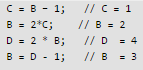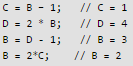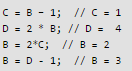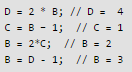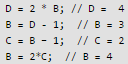There are 3 different possible values of B: 2, 3 and 4.

Test: Process- 1 - Question 13

Two processes X and Y need to access a critical section. Consider the following synchronization construct used by both the processes.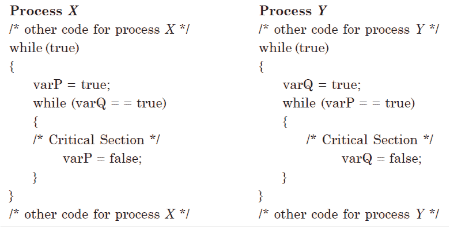Q. Here, varP and varQ are shared variables and both are initialized to false. Which one of the following statements is true?

Detailed Solution for Test: Process- 1 - Question 13

When both processes try to enter critical section simultaneously,both are allowed to do so since both shared variables varP and varQ are true.So, clearly there is NO mutual exclusion. Also, deadlock is prevented because mutual exclusion is one of the four conditions to be satisfied for deadlock to happen.Hence, answer is A.

Test: Process- 1 - Question 14

In a certain operating system, deadlock prevention is attempted using the following scheme. Each process is assigned a unique timestamp, and is restarted with the same timestamp if killed. Let Ph be the process holding a resource R, Pr be a process requesting for the same resource R, and T(Ph) and T(Pr) be their timestamps respectively. The decision to wait or preempt one of the processes is based on the following algorithm.

if T(Pr) < T(Ph)
then kill Pr
else wait

Q. Which one of the following is TRUE?

Detailed Solution for Test: Process- 1 - Question 14
1. This scheme is making sure that the timestamp of requesting process is always lesser than holding process
2. The process is restarted with same timestamp if killed and that timestamp can NOT be greater than the existing time stamp

From 1 and 2,it is clear that any new process coming having LESSER timestamp will be KILLED.So,NO DEADLOCK possible However, a new process will lower timestamp may have to wait  infinitely because of its LOWER timestamp(as killed process will also have same timestamp ,as it was killed earlier).STARVATION IS Definitely POSSIBLE So Answer is A

Test: Process- 1 - Question 15

A process executes the following segment of code :

for(i = 1; i < = n; i++)
fork ();

The number of new processes created is

Detailed Solution for Test: Process- 1 - Question 15

fork ();     // Line 1
fork ();     // Line 2
fork ();     // Line 3
.....till n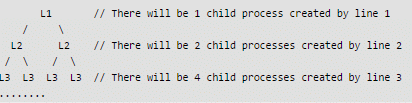We can also use direct formula to get the number of child processes. With n fork statements, there are always 2n – 1 child processes. Also see this post for more details.

Test: Process- 1 - Question 16

The semaphore variables full, empty and mutex are initialized to 0, n and 1, respectively. Process P1 repeatedly adds one item at a time to a buffer of size n, and process Prepeatedly removes one item at a time from the same buffer using the programs given below. In the programs, K, L, M and N are unspecified statements.

P1

while (1) {     K; P(mutex); Add an item to the buffer; V(mutex);     L; } P2 while (1) {    M; P(mutex); Remove an item from the buffer; V(mutex);     N; } The statements K, L, M and N are respectively

Detailed Solution for Test: Process- 1 - Question 16

Process P1 is the producer and process P2 is the consumer.
Semaphore ‘full’ is initialized to '0'. This means there is no item in the buffer. Semaphore ‘empty’ is initialized to 'n'. This means there is space for n items in the buffer.
In process P1, wait on semaphore 'empty' signifies that if there is no space in buffer then P1 can not produce more items. Signal on semaphore 'full' is to signify that one item has been added to the buffer.
In process P2, wait on semaphore 'full' signifies that if the buffer is empty then consumer can’t not consume any item. Signal on semaphore 'empty' increments a space in the buffer after consumption of an item.

Thus, option (D) is correct.

Please comment below if you find anything wrong in the above post.

Test: Process- 1 - Question 17

Consider the following two-process synchronization solution.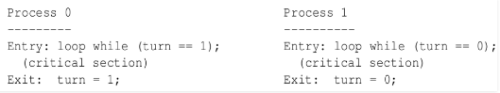The shared variable turn is initialized to zero. Which one of the following is TRUE?

Detailed Solution for Test: Process- 1 - Question 17

It satisfies the mutual excluision : Process P0 and P1 could not have successfully executed their while statements at the same time as value of ‘turn’ can either be 0 or 1 but can’t be both at the same time. Lets say, when process P0 executing its while statements with the condition “turn == 1”, So this condition will persist as long as process P1 is executing its critical section. And when P1 comes out from its critical section it changes the value of ‘turn’ to 0 in exit section and because of that time P0 comes out from the its while loop and enters into its critical section. Therefore only one process is able to execute its critical section at a time.

Its also satisfies bounded waiting : It is limit on number of times that other process is allowed to enter its critical section after a process has made a request to enter its critical section and before that request is granted. Lets say, P0 wishes to enter into its critical section, it will definitely get a chance to enter into its critical section after at most one entry made by p1 as after executing its critical section it will set ‘turn’ to 0 (zero). And vice-versa (strict alteration).

Progess is not satisfied : Because of strict alternation no process can stop other process from entering into its critical section.

Test: Process- 1 - Question 18

Consider a non-negative counting semaphore S. The operation P(S) decrements S, and V(S) increments S. During an execution, 20 P(S) operations and 12 V(S) operations are issued in some order. The largest initial value of S for which at least one P(S) operation will remain blocked is

Detailed Solution for Test: Process- 1 - Question 18

20-7 -> 13 will be in blocked state, when we perform 12 V(S) operation makes 12 more process to get chance for execution from blocked state. So one process will be left in the queue (blocked state) here i have considered that if a process is in under CS then it not get blocked by other process.

Test: Process- 1 - Question 19

Which of the following DMA transfer modes and interrupt handling mechanisms will enable the highest I/O band-width?

Detailed Solution for Test: Process- 1 - Question 19
• CPU  get highest bandwidth in transparent DMA and polling. but it asked for I/O bandwidth not cpu bandwidth so option (A) is wrong.
• In case of Cycle stealing, in each cycle time device send data then wait again after few CPU cycle it sends to memory . So option (B) is wrong.
• In case of Polling CPU takes the initiative so I/O bandwidth can not be high so option (D) is wrong .
• Consider Block transfer, in each single block device send data so bandwidth ( means the amount of data ) must be high.

This makes option (C) correct.

Test: Process- 1 - Question 20

In the working-set strategy, which of the following is done by the operating system to prevent thrashing?

1. It initiates another process if there are enough extra frames.
2. It selects a process to suspend if the sum of the sizes of the working-sets exceeds the total number of available frames.
Detailed Solution for Test: Process- 1 - Question 20

According to concept of thrashing,

• I is true because to prevent thrashing we must provide processes with as many frames as they really need "right now".If there are enough extra frames, another process can be initiated.
• II is true because The total demand, D, is the sum of the sizes of the working sets for all processes. If D exceeds the total number of available frames, then at least one process is thrashing, because there are not enough frames available to satisfy its minimum working set. If D is significantly less than the currently available frames, then additional processes can be launched.

## GATE Computer Science Engineering(CSE) 2023 Mock Test Series

150 docs|215 tests
 Use Code STAYHOME200 and get INR 200 additional OFF Use Coupon Code
Information about Test: Process- 1 Page
In this test you can find the Exam questions for Test: Process- 1 solved & explained in the simplest way possible. Besides giving Questions and answers for Test: Process- 1, EduRev gives you an ample number of Online tests for practice

## GATE Computer Science Engineering(CSE) 2023 Mock Test Series

150 docs|215 tests